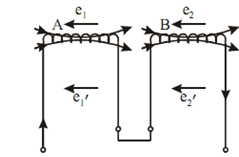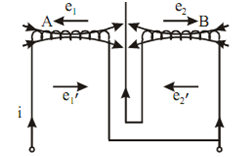## Inductances in Series and Parallel Assignment Help

Assignment Help: >> Inductance - Inductances in Series and Parallel

Inductances in Series and Parallel:

Inductances in Series

(a) Let the two coils be linked in series such that their fluxes are additive,Figure: Series Inductors

Self induced emf in A = e1 = - L1(di /dt)

Mutually induced emf in A due to change in B = e1′ = - M (di/ dt)

Self induced emf in     B2 = e2 = - L2   (di/ dt)

Mutually induced emf in B because of change in A2 = e2′ = - M  (di/ dt)

Total induced emf  =- di /dt  (L1 + L2 + 2M )

If L refers to the equivalent inductance then total induced emf in that single coil is given as,

=- L di /dt

From Eqs. (22) and (23) we get

L = (L1 + L2  + 2M )

(b)       While coils are so connected that their fluxes are in opposite direction,Figure: Inductor in Series Opposition

Now,

e1=-L1 (di/dt) and e1' = M (di/dt)

e2 = L2 (di/dt) and e2' = M (di/dt)

Total induced emf  =-(di/dt)  (L1 + L2 - 2M )

∴ Equivalent inductance L = L1 + L2  - 2M